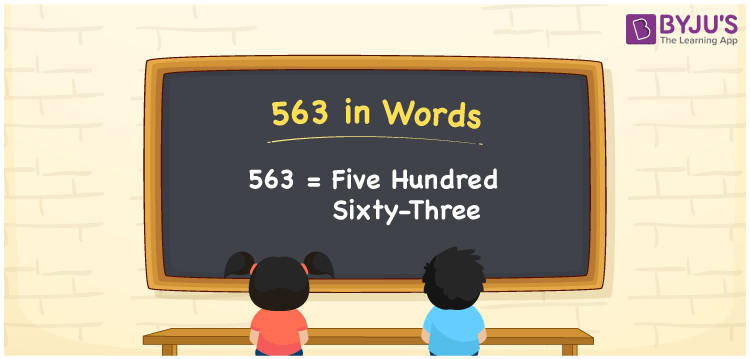# 563 in words

563 in wordsi s written as Five Hundred and Sixty Three. 563 represents the count or value. The article on Counting Numbers can give you an idea about count or counting. The number 563 is used in expressions that relate to money, distance, length, year and others. Let us consider an example for 563. ”They took Five Hundred and Sixty Three steps.”

 563 in words Five Hundred and Sixty Three Five Hundred and Sixty Three in Numbers 563

## 563 in English Words## How to Write 563 in Words?

We can convert 563 to words using a place value chart. The number 563 has 3 digits, so let’s make a chart that shows the place value up to 3 digits.

 Hundreds Tens Ones 5 6 3

Thus, we can write the expanded form as:

= 5 × Hundred + 6 × Ten + 3 × One

= 5 × 100 + 6 × 10 + 3 × 1

= 563

= Five Hundred and Sixty Three.

563 is the natural number that is succeeded by 562 and preceded by 564.

563 in words – Five Hundred and Sixty Three.

Is 563 an odd number? – Yes.

Is 563 an even number? – No.

Is 563 a perfect square number? – No.

Is 563 a perfect cube number? – No.

Is 563 a prime number? – Yes.

Is 563 a composite number? – No.

## Solved Example

1. Write the number 563 in expanded form

Solution: 5 × 100 + 6 × 10 + 3 × 1

We can write 563 = 500 + 60 + 3

= 5 × 100 + 6 × 10 + 3 × 1

## Frequently Asked Questions on 563 in words

Q1

### How to write 563 in words?

563 in words is written as Five Hundred and Sixty Three.
Q2

### Is 563 a perfect square number?

No. 563 is not a perfect square number.
Q3

### Is 563 a prime number?

Yes. 563 is a prime number.